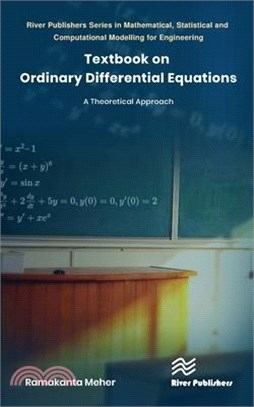TOP
【即將送完！限量贈「百樂魔擦印章」1+1】日本最強商品開發者終於出書！企劃祕技大公開！滿額折

# Textbook on Ordinary Differential Equations: A Theoretical Approach

定  價：NT\$ 6705 元
若需訂購本書，請電洽網路書店客服02-25006600[分機130、131]。

## 主題書展

商品簡介
商品簡介

Many scientific and real-world problems that occur in science, engineering, and medicine can be represented in differential equations. There is a vital role for differential equations in studying the behavior of different types of real-world problems. Thus, it becomes crucial to know the existence uniqueness properties of differential equations and various methods of finding differential equation solutions in explicit form. It is also essential to know different kinds of differential equations in terms of eigenvalues, termed eigenvalue problems, and some special functions used in finding the solution to differential equations. The study of nonlinear problems also plays a significant role in different real-world situations. There is a necessity to know the behavior of solutions of nonlinear differential equations. Still, there are very few forms of differential equations whose solution can be found in explicit form. For the differential equations whose solutions cannot be found in explicit form, one has to study the properties of solutions of the given differential equation to guess an approximate solution of it. This book aims to introduce all the necessary topics of differential equations in one book so that laymen can easily understand the subject and apply it in their research areas. The novel approach used in this book is that I have introduced different analytical methods for finding the solution of differential equations with sufficient theorems, corollaries, and examples, and the geometrical interpretations in each topic.

This textbook is intended to study the theory and methods of finding the explicit solutions to differential equations, wherever possible, and in the absence of finding explicit solutions. It is intended to study the properties of solutions to the given differential equations. This book is based on syllabi of the theory of differential equations prescribed for postgraduate students of mathematics and applied mathematics in different institutions and universities of India and abroad. This book will be helpful for competitive examinations as well.

## 購物須知

外文書商品之書封，為出版社提供之樣本。實際出貨商品，以出版社所提供之現有版本為主。部份書籍，因出版社供應狀況特殊，匯率將依實際狀況做調整。

無庫存之商品，在您完成訂單程序之後，將以空運的方式為你下單調貨。為了縮短等待的時間，建議您將外文書與其他商品分開下單，以獲得最快的取貨速度，平均調貨時間為1~2個月。

為了保護您的權益，「三民網路書店」提供會員七日商品鑑賞期(收到商品為起始日)。

若要辦理退貨，請在商品鑑賞期內寄回，且商品必須是全新狀態與完整包裝(商品、附件、發票、隨貨贈品等)否則恕不接受退貨。

優惠價：100 6705
若需訂購本書，請電洽網路書店客服02-25006600[分機130、131]。